CS 5319 Advanced Discrete Structure (Fall 2010)

General Information

 Lecturer:   Wing-Kai Hon  韓永楷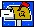wkhon @ cs.nthu.edu.tw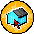EECS 741

 Tutor:   Wisely Ku  古宗翰thku @ cs.nthu.edu.tw

 Meeting Times: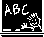Mon 1310—1500, Thu 1310—1400

 Announcements Final Exam is Coming! Date:    Jan 10, 2011 (2 hours) Scope: Notes 11 to 17, HW 6 Dec 16: Lecture 17 [pdf] posted Dec 20: Homework 6 [pdf] posted Dec 23: Lecture 18 [pdf] posted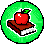Course Materials

 Lecture Topics Related Files 0 Overview [pdf] 1 Permutations and Combinations I   (Basics) [pdf] 2 Permutations and Combinations II  (Distribution of Objects) [pdf] 3 Generating Functions I  (Introduction, GF for Combinations) [pdf] 4 Generating Functions II (EGF for Permutations, Distribution of Objects) [pdf] 5 Recurrence Relations I  (Introduction, Linear Recurrence, Solving by GF) [pdf] 6 Recurrence Relations II (Special Form, Two Indices) [pdf] 7 Methods of Proving [pdf] 8 Number Theory I   (Divisibility, GCD, Fundamental Theorem of Arithmetic) [pdf] 9 Number Theory II  (Modular Arithmetic, Euler Function) [pdf] 10 Number Theory III (RSA Cryptosystem) [pdf] 11 Group Theory I   (Groups and Subgroups) [pdf] 12 Group Theory II  (Generators and Lagrange’s Theorem) [pdf] 13 Group Theory III (Permutation Group, Burnside’s Theorem) [pdf] 14 Group Theory IV (Group Codes) [pdf] 15 Automata Theory I  (DFA, Pumping Lemma) [pdf] 16 Automata Theory II (NFA, Equivalence of DFA and NFA) [pdf] 17 NP-Completeness [pdf] 18 Approximation Algorithms [pdf]

 Assignment Topics Related Files 1 Permutations and Combinations [pdf] 2 Generating Functions [pdf] 3 Recurrence Relations [pdf] 4 Methods of Proving [pdf] 5 Number Theory [pdf] 6 Group Theory and Theory of Computation [pdf]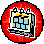Teaching Plan

 1 Permutations and Combinations Lecture 01 to Lecture 02 2 Generating Functions Lecture 03 to Lecture 04 3 Recurrence Relations Lecture 05 to Lecture 06 4 Methods of Proving Lecture 07 5 Number Theory Lecture 08 to Lecture 10 6 Group Theory Lecture 11 to Lecture 14 7 Automata Theory Lecture 15 to Lecture 16

Last updated:  December 23,  2010